# statsmodels.nonparametric.kernel_density.KDEMultivariateConditional.imse¶

KDEMultivariateConditional.imse(bw)[source]

The integrated mean square error for the conditional KDE.

Parameters: bw: array_like The bandwidth parameter(s). CV: float The cross-validation objective function.

Notes

For more details see pp. 156-166 in [R15]. For details on how to handle the mixed variable types see [R16].

The formula for the cross-validation objective function for mixed variable types is: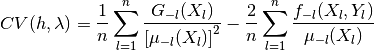where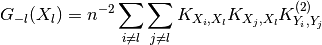where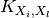is the multivariate product kernel and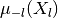is the leave-one-out estimator of the pdf.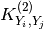is the convolution kernel.

The value of the function is minimized by the _cv_ls method of the GenericKDE class to return the bw estimates that minimize the distance between the estimated and “true” probability density.

References

 [R15] (1, 2) Racine, J., Li, Q. Nonparametric econometrics: theory and practice. Princeton University Press. (2007)
 [R16] (1, 2) Racine, J., Li, Q. “Nonparametric Estimation of Distributions with Categorical and Continuous Data.” Working Paper. (2000)